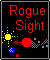#Variables

The movement of the 12th planet, due to gravitational attraction between the binary stars can be predicted by a hyperbolic exponential function of velocity vs time. To simplify matters we will use standard formulas and transpose the variables to derive an equation suited for our needs. Since the velocity of the 12th planet is increasing exponentially, the equation for population growth can be used. This equation, which can be referenced in any Calculus book, is Y (final) = Y (initial) * e ^ [ (k) * (t)]. Y (final) and Y (initial) respectively, are the final and initial totals of population. The letter e is the exponential function, t is time interval and k the rate of which birth increases or decreases. The symbol ^ represents variables that follow are exponents of e.

For our purposes, we will substitute the variables in the population equation with a new set of variables to solve our problem. Since the formula, distance = velocity (rate of speed) * time is similar to population = rate of birth * time. We will use this as a base equation. Transposing, Distance (final) = Distance (initial) * e ^ [(k) * (t)] where (k) is the rate at which gravity accelerates or decelerates the velocity of the 12th planet and (t) represents the interval of time between initial and final distances. The hyperbolic curve we are examining, is the latter part of a bisected hyperbolic cosine curve representing total movement from the Dark One to the Sun. The time interval between two reference points, the Sun and the deflection point from the midpoint in the orbital path of the 12th planet will be used to find the rate of acceleration or (k) rate.

Distance (final) is equal to 18.724 times the distance from the Sun to Pluto divided by 2. This is the distance from the midpoint between the binary stars to the Sun. This is equal to 9.362 Sun/Pluto units. Using what we are given, the 12th planet passes a deflection point at 2.5196 times the distance of Sun to Pluto and passes the Sun some 9.7 weeks later. This point is where repulsion particles emanating from the Sun, apply a sufficient opposing force to the Sun's gravitational attraction, altering the orbit of the 12th planet. The new orbital track is initiated, as a shallow clockwise parabolic curve slightly down and away from the Sun. Distance (initial) is at (18.724 S-P units / 2) - 2.5196 S-P units, the deflection point, which yields 6.8424 S-P units from the midpoint.

The variable (k), the rate of increase in velocity or acceleration is unknown. The variable (t) is equal to the time interval of 9.7 weeks for the 12th planet to move between the deflection point, Distance (initial) and Distance (final), the Sun. Solving for (k), divide both sides of the equation [Distance(Final) = Distance(initial) * e ^ ((t) * (k))] by Distance(initial) and multiply both sides by the function of LN(natural logarithm base e). This yields LN[Distance(final) / Distance(initial)] = (k)(t). Proceeding, divide both sides by (t) and substitute values in for variables.

Offered by Robert.